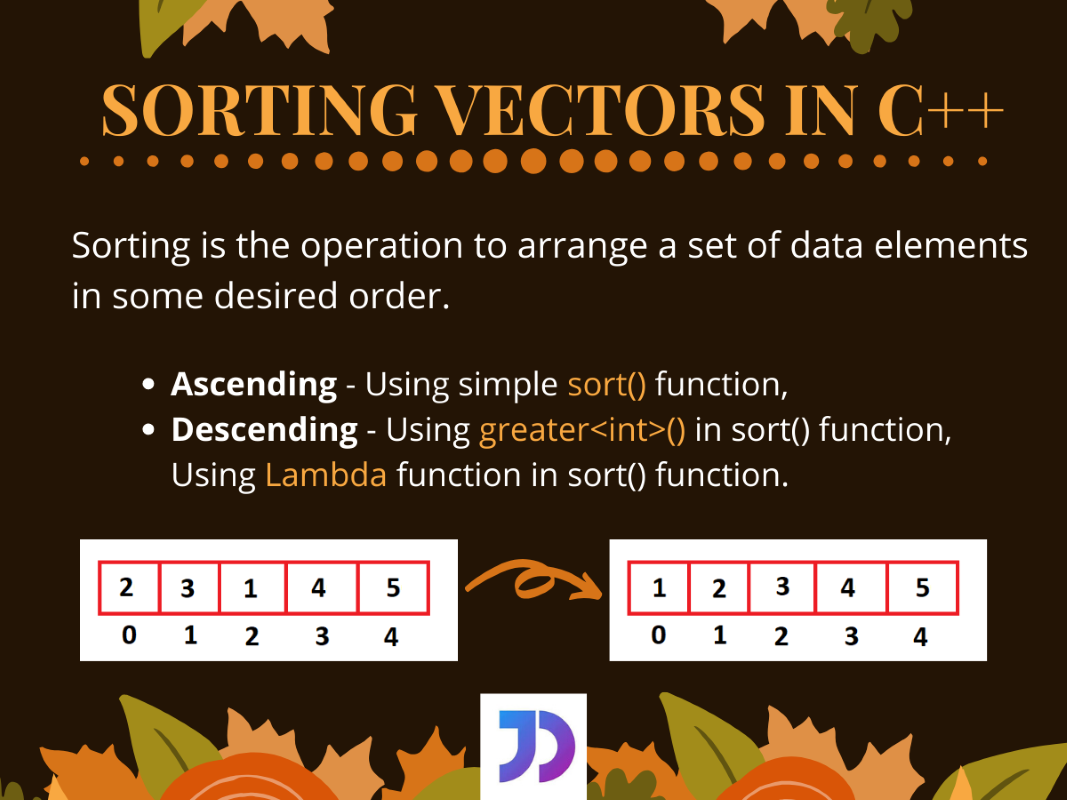# Sorting a Vector in C++

Published on August 3, 2022By SnehWhile we believe that this content benefits our community, we have not yet thoroughly reviewed it. If you have any suggestions for improvements, please let us know by clicking the “report an issue“ button at the bottom of the tutorial.

## Introduction

In this tutorial, we are going to focus on Sorting a Vector in C++.

Sorting is one of the vastly performed operations in any programming language. Similarly, in C++ too, there are several algorithms following which we can sort any data structure.

For vectors, in particular, we can perform sorting operations in any order(ascending or descending).

## Sorting a Vector in C++ in Ascending order

A vector in C++ can be easily sorted in ascending order using the `sort()` function defined in the `algorithm` header file.

The `sort()` function sorts a given data structure and does not return anything. The sorting takes place between the two passed iterators or positions. The third parameter determines the order in which the elements are going to be compared.

By default, for not passing the third parameter, the function considers it to be the `std::less<int>()` function. This function returns true or false on the basis of comparing two arguments, whether the first one is less than the other.

So, now let us see how we can sort a vector in C++(ascending order).

``````#include<iostream>
#include<vector>
#include<algorithm>
using namespace std;
int main()
{
//vector initialisation
vector<int> vec {5, 4, 3, 2, 1};

cout<<"Before sorting vector : ";
for(auto i=vec.begin(); i<vec.end(); i++)
{
cout<<" "<<*i;
}

std::sort(vec.begin(),vec.end());//Sorting the vector

cout<<"\n\nAfter sorting vector : ";
for(auto i=vec.begin(); i<vec.end(); i++)
{
cout<<" "<<*i;
}
return 0;
}
``````

Output:

``````Before sorting vector :  5 4 3 2 1

After sorting vector :  1 2 3 4 5
``````

## Sorting a Vector in C++ in Descending Order

As we said earlier, the third argument for the `sort()` function in C++ determines the order of sorting. So, we can define functions in it to sort any vector in our desired order(descending in this case).

### 1. Using greater<int>() in sort()

Similar to the `less<int>()` function, the `greater<int>()` function returns a bool value as true or false but in the opposite sense. If the first argument is greater than the second one, the function returns true and false if the above condition is false.

Let us see how we can use it to get a sorted vector in descending order.

``````#include<iostream>
#include<vector>
#include<algorithm>
using namespace std;
int main()
{
//vector initialisation
vector<int> vec { 2,4,6,8,10 };

cout<<"Before sorting vector : ";
for(auto i=vec.begin(); i<vec.end(); i++)
{
cout<<" "<<*i;
}

std::sort(vec.begin(),vec.end(), greater<int>());//Sorting the vector using greater<int>() function

cout<<"\n\nAfter sorting vector : ";
for(auto i=vec.begin(); i<vec.end(); i++)
{
cout<<" "<<*i;
}
return 0;
}
``````

Output:

``````Before sorting vector :  2 4 6 8 10

After sorting vector :  10 8 6 4 2
``````

### 2. Using Lambda Expression in sort()

Since C++11, the use of lambda expressions was introduced to C++ programming. They are nothing but simple one-line functions, which do not need declaration or even require to specify their return type.

Hence, we can use our own defined lambda expression to determine the order of sorting by the `sort()` function. This can be done by defining the one-line expression as the third parameter to the `sort()` function. Let see how

``````#include<iostream>
#include<vector>
#include<algorithm>
using namespace std;
int main()
{
//vector initialisation
vector<int> vec { 11,22,33,44,55 };

cout<<"Before sorting vector : ";
for(auto i=vec.begin(); i<vec.end(); i++)
{
cout<<" "<<*i;
}

std::sort(vec.begin(),vec.end(), [](int &a, int &b){ return a>b; });
//Sorting the vector using user-defined lambda expression(return type bool)

cout<<"\n\nAfter sorting vector : ";
for(auto i=vec.begin(); i<vec.end(); i++)
{
cout<<" "<<*i;
}
return 0;
}
``````

Output:

``````Before sorting vector :  11 22 33 44 55

After sorting vector :  55 44 33 22 11
``````

Here, the expression `a>b` is used to compare two passed arguments from the vector. As we can see from the output for the above code, the vector gets sorted in descending order as desired.

## Conclusion

So, in this article, we learned about sorting a vectors in C++, in both ascending and descending order. For any further questions related to this topic, feel free to use the comments below.

Click below to sign up and get \$200 of credit to try our products over 60 days!

### Popular Topics##### Hollie's Hub for Good

Working on improving health and education, reducing inequality, and spurring economic growth? We’d like to help.##### Become a contributor

You get paid; we donate to tech nonprofits.

##### Welcome to the developer cloud

DigitalOcean makes it simple to launch in the cloud and scale up as you grow – whether you’re running one virtual machine or ten thousand.© 2023 DigitalOcean, LLC.